# Team:Glasgow/Modeling

Bubble Test Page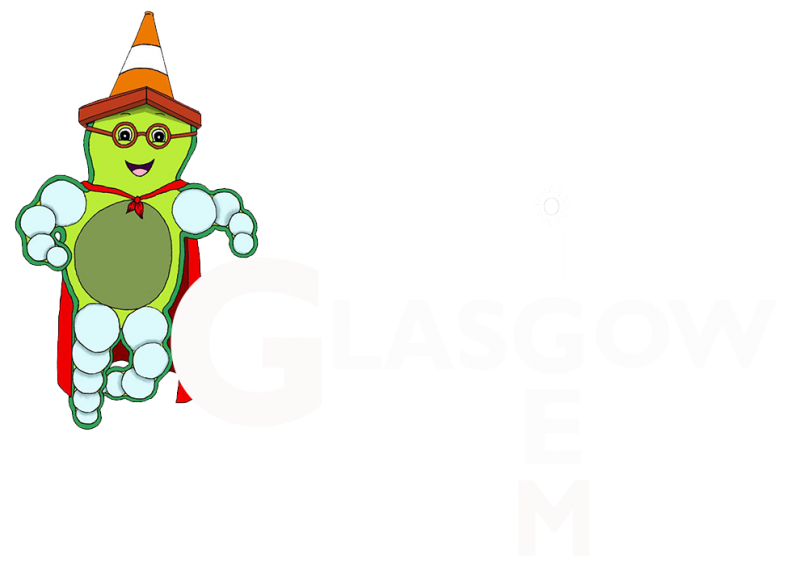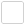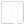## Section 1: Modelling of Bacteria Random Walk

Firstly, we created a very basic 2D model of a flagella propelled bacterium. This was heavily based on the “random walk” model we mentioned previously, only we introduced a small degree of order, based on a more extensive and all-encompassing model created by Dillon, Fauci and Gaver in 19951.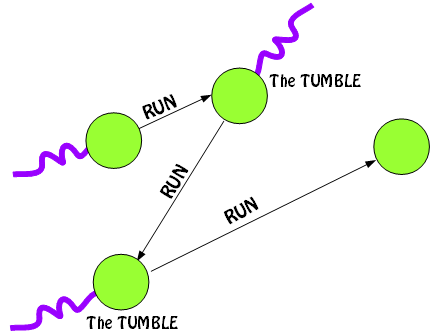Figure 1: Basic Run and Tumble

In order to simplify the model, we made a number of assumptions. These are:

• Tumbling is instantaneous
• Chemotaxic gradient is not a factor and so the run time has an element of randomness
• An E.coil cell can be represented as a sphere
• Speed is constant (20μms-1)2

The movement of a bacteria through a medium is described thus:
1. The bacteria is moving at a random angle at a certain speed.
2. After a certain time (the “run” time), the bacteria reorientates itself (the “tumble”),
and sets off at a different angle. This run time can be influenced by the chemotaxic gradient,
if present, which will induce directionality in the motion.

The images below describe how the run times are influenced: if the bacteria is on a path towards the "food", it is unlikely to change direction.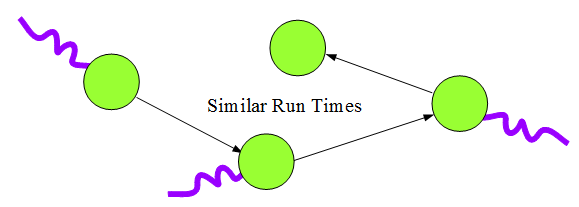Figure 2: Bacterial movement with no chemotaxic gradient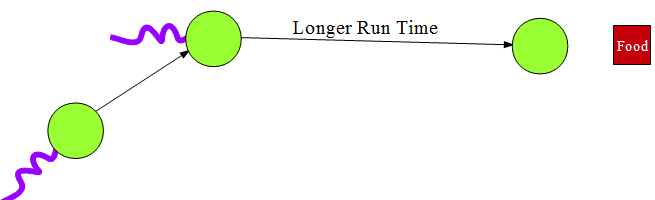Figure 3:Alteration of bacterial random walk with chemotaxic gradient present

Based on the previous research, we decided on some distributions for our models' random parameters.
Tumble Angle

• Normal Distribution
• Mean: 68 degrees
• Standard Deviation: 36 degrees

Run Time

• Exponential Distribution
• Mean: 1 second

The angle would be either added or subtracted from the previous position. The speed was set at a constant 20μs-1, and the run time would be selected from the exponential distribution(as chemotaxis was not a factor). Given angle, speed, and time, new x and y coordinates were calculated, and a line plotted to show the path. This process can be repeated for any number of steps to show the theoretical path of a bacterium.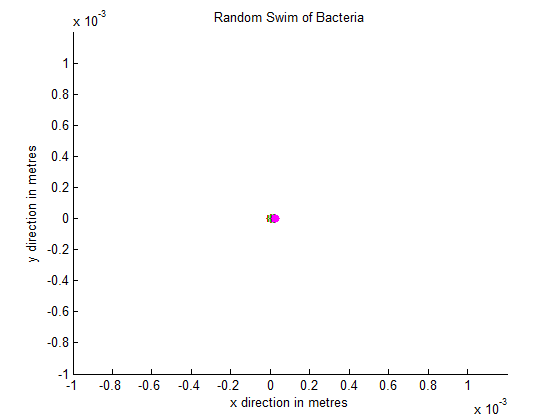Figure 5: Giff displaying path of 10 bacteria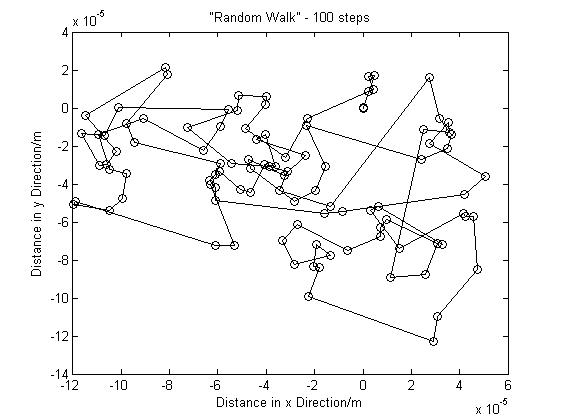Figure 4: a 100 step Random Walk Simulation

This is the result of a MATLAB simulation using the angle and run time distributions stated above. The gif shows the path of 10 different bacterium, and how they move out from a central point. Each makes 300 steps - so please note than the graphs are not directly comparable due to the difference in scale bacteria will travel further in 300 steps than 100. Rather, they should help display the randomness of the motion. Roughly, the gif is 10x faster than normal movement (assuming the mean run time is 1 second).

The simulation we'd created did, of course, have a significant element of randomness. Below is an example of a run that provided us with a random (but strangely familiar) bacterial path.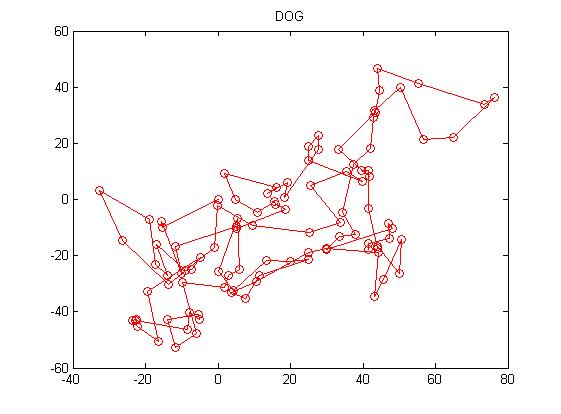Figure 6: "Random Dog"

Having obtained a reasonable working model of a bacterium's natural movement, we could now investigate the effect of the buoyancy provided by the gas vesicles we'd be introducing. With this information, we could make informed decisions about the direction of the project.

References
1. Dillon,R. Fauci,L. Gaver,D. A Microscale Model of Bacterial Swimming, Chemotaxis and Substrate Transport.Journal of Theoretical Biology.1995. Vol 177(4). p 325-340.DOI: 10.1006/jtbi.1995.0251. (accessed July 2014)
2. Walsby, AE. Gas Vesicles. Microbiological Reviews. 1994. Volume 58. p 138.http://www.ncbi.nlm.nih.gov/pmc/articles/PMC372955/.(accessed June 2014)

Edit